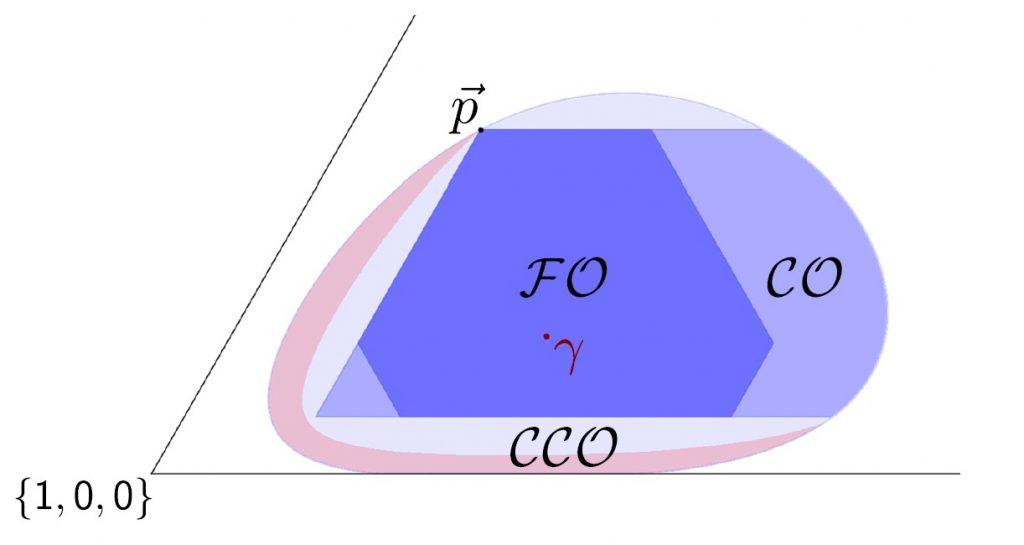# Fundamental Limits on Correlated Catalytic State Transformations

Quantum state transformation is a fundamental problem in quantum resource theories. Resource theories offer a general framework to quantify the usefulness of quantum states and their inconvertibility under free operations. A resource theory is defined by a subset of quantum states called free states and a set of free operations with the property that map free states into free states. In this picture, the free states do not contain any resource and the free operations cannot create a resource state when acting on a free state. A prominent example is entanglement theory where entanglement is the resource, the free states are the separable states and the free operations are the local operations and classical communications (LOCC). Given a fixed state $\rho$, we call $\mathcal{FO}(\rho)$ the set of all $\rho'$ that can be reached from $\rho$ using free operations.

A natural question is whether a catalyst could enhance quantum state transformation. A catalyst is an ancillary system that assists the transformation but it is left unchanged at the end of the process. The term catalyst is borrowed from chemistry. Here, a catalyst is a substance that enables or increases the rate of a chemical reaction without itself changing during the process. We call $\mathcal{CO}(\rho)$ the set of all states $\rho'$ for which there exists a catalyst and a free operation which maps $\rho$ into $\rho'$ leaving the catalyst unchanged. More recently, a further relaxation has been studied where correlations between the catalyst and the target state after the transformation are allowed and can be used as a resource to enhance catalytic transformations. Similarly, we call $\mathcal{CCO}(\rho)$ the set of all states $\rho'$ for which a catalytic transformation with residual correlations from $\rho$ exists. In this case, at the end of the process, there are non-zero residual correlations between the catalyst and the output state $\rho'$. We show below an example of these sets for the classical resource theory of athermality.Example of the sets \mathcal{FO} , \mathcal{CO} and \mathcal{CCO} for classical resource theory of athermality. In this resource theory, the thermal state \gamma=e^{-\beta H}/Z is the only free state of the theory. Here, \beta is the inverse temperature, H the Hamiltonian and Z the normalisation factor. Any other state that is outside the thermal equilibrium state is considered as resourceful. The free operations are the Gibbs preserving operations, i.e. all the operations that keep the Gibbs state invariant. In classical resource theory of athemality we consider only classical states, i.e. states that are diagonal in the eigenbasis of the Hamiltonian. We fixed the input state \vec{p}=\{2/3, 1/12, 3/12\} and \gamma = \{7/10, 2/10, 1/10\}. We show one corner of the probability simplex (which is a triangle in this case). Each point in the triangle corresponds to a (classical) state of a three-dimensional system. To transform the input state \vec{p} into the states in the red region, we need a catalyst whose resources diverge as the correlations vanish.

In our work we study correlated catalytic transformations. Our main contribution is to find a trade-off between the residual correlation between the system and the catalyst at the end of the process and the resource of the catalyst that we need to prepare to perform the transformation. We find that for a large class of transformations, a small residual correlation between catalyst and target state implies that the catalyst needs to be highly resourceful. In fact, the resources required diverge in the limit of vanishing residual correlation. In the figure above, we show in red the set of output states that need a catalyst whose resources diverge as the correlations vanish.

Our work was presented at AQIS21, BIID’9, and QIP22 and it was published in Physical Review Letters.

This entry was posted in Uncategorized. Bookmark the permalink.